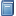Buchempfehlung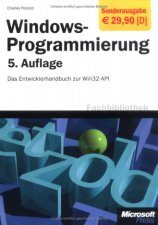Windows-Programmierung. Das Entwicklerhandbuch zur WIN32-API
"Der" Petzold, das über 1000 Seiten starke Standardwerk zum Win32-API - besonders nützlich u. a. bei der GUI-Programmierung in FreeBASIC! [Mehr Infos...]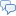FreeBASIC-Chat
Es sind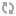Benutzer im FreeBASIC-Chat online.
(Stand:  )

# fb:porticula NoPaste

## GameOfLife.bi

 Uploader: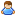OneCypher Datum/Zeit: 13.10.2009 11:04:28
Hinweis: Dieser Quelltext ist Bestandteil des Projekts GuiPtr, zu dem es auf FreeBASIC-Portal.de eine Projektseite gibt.

```#include once "GuiPtr.bi" #include once "GuiWindow.bi" 'Game Of Life: Type GameOfLife     VWindow as GuiWindow = GuiWindow(0,0,136,176,"It's living...")     ViewField as any ptr = imageCreate(128,128,RGB(0,0,0))     GolField(1 to 2, 0 to 129, 0 to 129) as ubyte     AField as ubyte = 1     BField as ubyte = 2     FPS as integer     LastFrame as double     FrameCounter as integer     ImageRotation as integer     declare sub CalcOneStep()     declare sub ShowGOLBuffer()     declare sub RandomizeAll()     declare sub EraseAll()     declare function CountAround(x as integer ,y as integer) as integer     declare constructor (left as integer, top as integer,title as string) end type Sub RedrawVWindow(GO as any ptr)     dim G as GameOfLife ptr = GO     dim w as integer = G->VWindow.Object->width -8     dim h as integer = G->VWindow.Object->height -48     'dump str(w) & " " & str(h)     Dim TmpImage as any ptr = imagecreate(w,h,RGB(0,0,0))     G->ShowGOLBuffer     rotozoom_alpha2( TmpImage, G->ViewField, w / 2, h / 2, G->ImageRotation, w / 128, h / 128)     Put G->VWindow.Object->Buffer, (4,46), TmpImage,PSET     imageDestroy TmpImage end sub Constructor GameOfLife(left as integer, top as integer, title as string)     VWindow.Object->ClassName = "GameOfLife"     VWindow.Object->Left = left     VWindow.Object->top = top     VWindow.Object->MyObject = @This     VWindow.Object->DrawPriority = 0     VWindow.Object->PublicEvents->OnDraw = @RedrawVWindow     VWindow.title = title end constructor Sub GameOfLife.ShowGOLBuffer()     for x as integer = 1 to 128         for y as integer = 1 to 128            if GolField(AField,x,y) = 1 then                pset ViewField,(x,y),RGB(255,255,255)             else                pset ViewField,(x,y),RGB(0,0,0)             end if         next     next end sub sub GameOfLife.EraseAll()     for i as integer = 1 to 128 * 128 / 2         GolField(AField,int(RND*127)+1,int(RND*127)+1) = 0     next end sub sub GameOfLife.RandomizeAll()     dim tx as integer, ty as integer     for i as integer = 1 to 128 * 128 / 2         tx = int(RND*127)+1         ty = int(RND*127)+1         GolField(AField,tx,ty) = 1     next end sub Function GameOfLife.CountAround(x as integer ,y as integer) as integer     'Drumrum zählen     Dim c as integer     for dx as integer = -1 to 1         for dy as integer = -1 to 1             if GolField(AField, x+dx,y+dy) = 1 then C = C +1         next     next     return C end function Sub GameOfLife.CalcOneStep()     'Ränder Spiegeln     dim tmp as ubyte     Dim pixdata As Any Ptr, pitch As Integer     Dim As UInteger Ptr p     if LastFrame = 0 then LastFrame = Timer     for x as integer = 1 to 128         GolField(AField,x,0) = GolField(AField,x,128)         GolField(AField,0,x) = GolField(AField,128,x)         GolField(AField,x,129) = GolField(AField,x,1)         GolField(AField,129,x) = GolField(AField,1,x)     next     'Einmal übers feld laufen...     for x as integer = 1 to 128         for y as integer = 1 to 128             'Dump "x" & x & " y" & y             GolField(BField, x,y) = GolField(AField, x,y)             tmp = CountAround(x,y)             if tmp = 3 then GolField(BField, x,y) = 1             if tmp < 3 then GolField(BField, x,y) = 0             if tmp > 4 then GolField(BField, x,y) = 0         next     next     swap AField, BField     FrameCounter += 1     If LastFrame + 2 <= timer then         FPS = FrameCounter / 2         FrameCounter = 0         LastFrame = Timer     end if end sub```